## 背景

1. 在存储空间上，相较于 FLOAT 的 32 bits 的大小，量化值占用的空间更小。
2. 在性能上，各类计算设备对量化值的计算能力要高于 FLOAT 的计算能力。

$Q(r)=Int(r/S)+Z$ 

r‘=S(Q(r)−Z)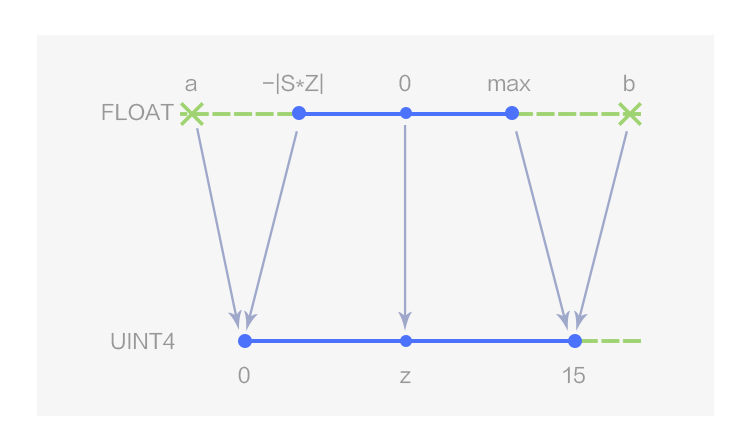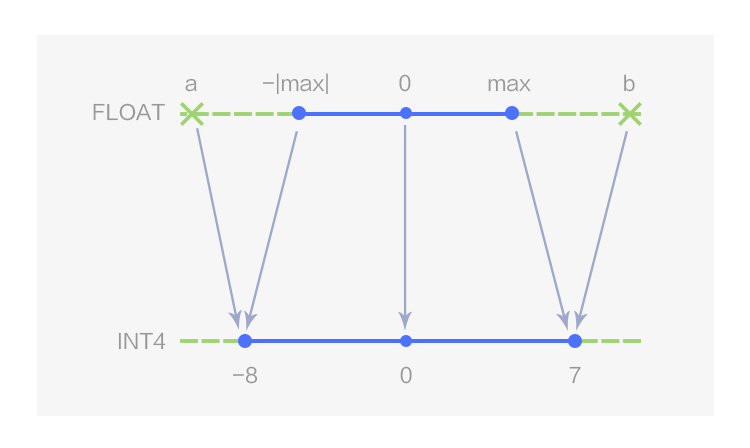## 缓解精度下降

FP32 原始计算一次卷积输出结果的公式：

$O = \sum_{i=0}^{Wn}(I_{i}\times W_{i}) + bias$

$Q(O) = \frac{\sum_{i=0}^{Wn}((Q(I_{i}) - Z_{I})\times S_{I}\times (Q(W_{i}) - Z_{W})\times S_{W})}{S_{O}}+Z_{O} + bias$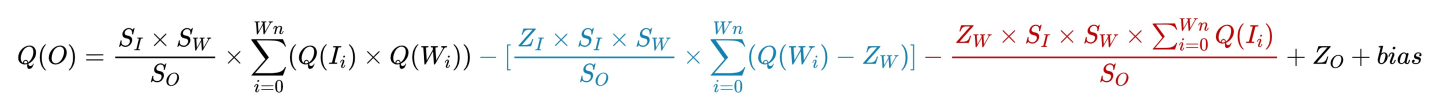1. 黑色表示无论输入/输出以及 weights 数据类型如何选择，一定有的计算量。因为无法避免，所以不用考虑这部分的数据特性。
2. 蓝色表示可以在推理前计算好的数据
3. 红色表示必须在推理时才能计算的数据。

• 全用 INT4 时，即 $Z_{I}$$Z_{W}$ 均等于 0， 计算量最小，只有黑色部分公式。
• 输入/输出用 UINT4，weights 用 INT4，即 $Z_{I}$ 不等于 0，$Z_{W}$ 等于 0 时，会增加蓝色公式部分的计算量，但是这个部分是可以提前运算好的，对整体计算时间影响不大。
• weights 用 UINT4，即 $Z_{W}$ 不等于 0 时， 会增加红色公式部分的计算量，会对整体的计算时间带来较大影响。

## 提升模型性能

• conv 计算非常多，占用了全图 80% 以上的运算时间。

### 算子融合优化

MegEngine 通过对计算图进行扫描匹配，并将匹配到的图结构替换为优化后的图结构。ResNet-50 模型所用的两种 pass 转换如下图所示: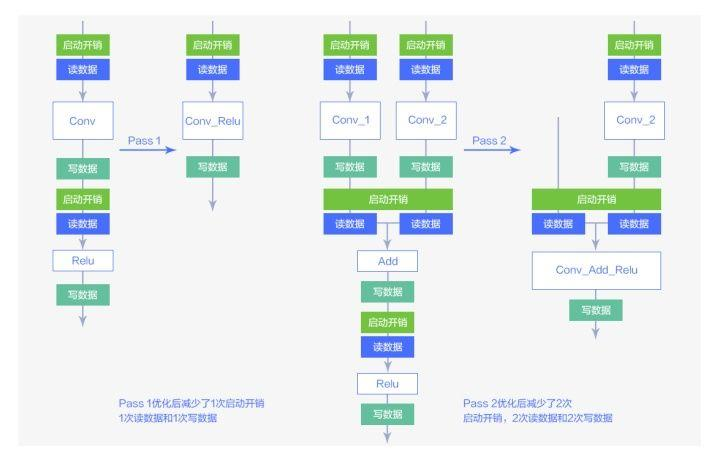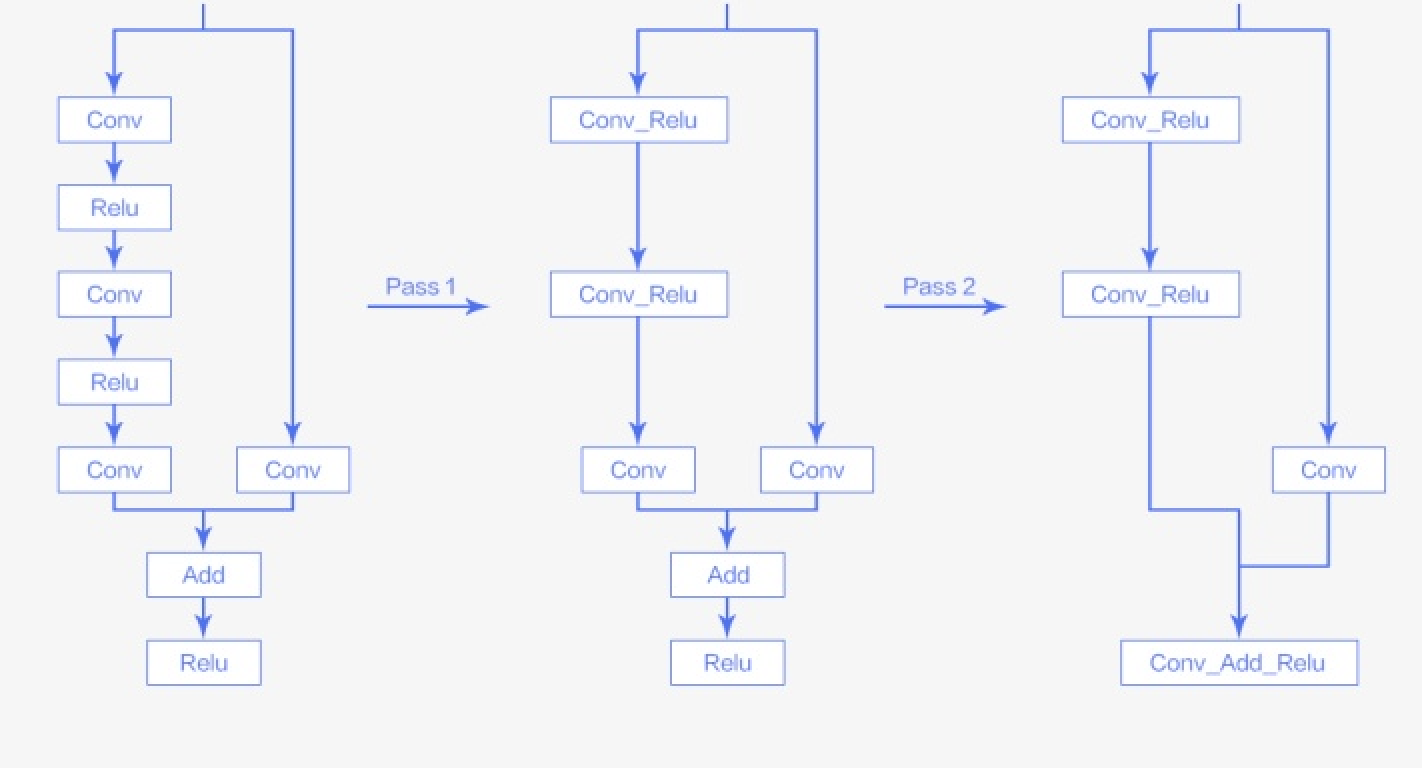### conv 算子优化

conv 采用 implicit gemm 算法并通过 mma 指令调度 tensor core 进行计算加速。 顾名思义，implicit gemm就是将 conv 运算转换为矩阵乘的一种算法，是对 img2col 的算法的改进，传统的 img2col 算法如下：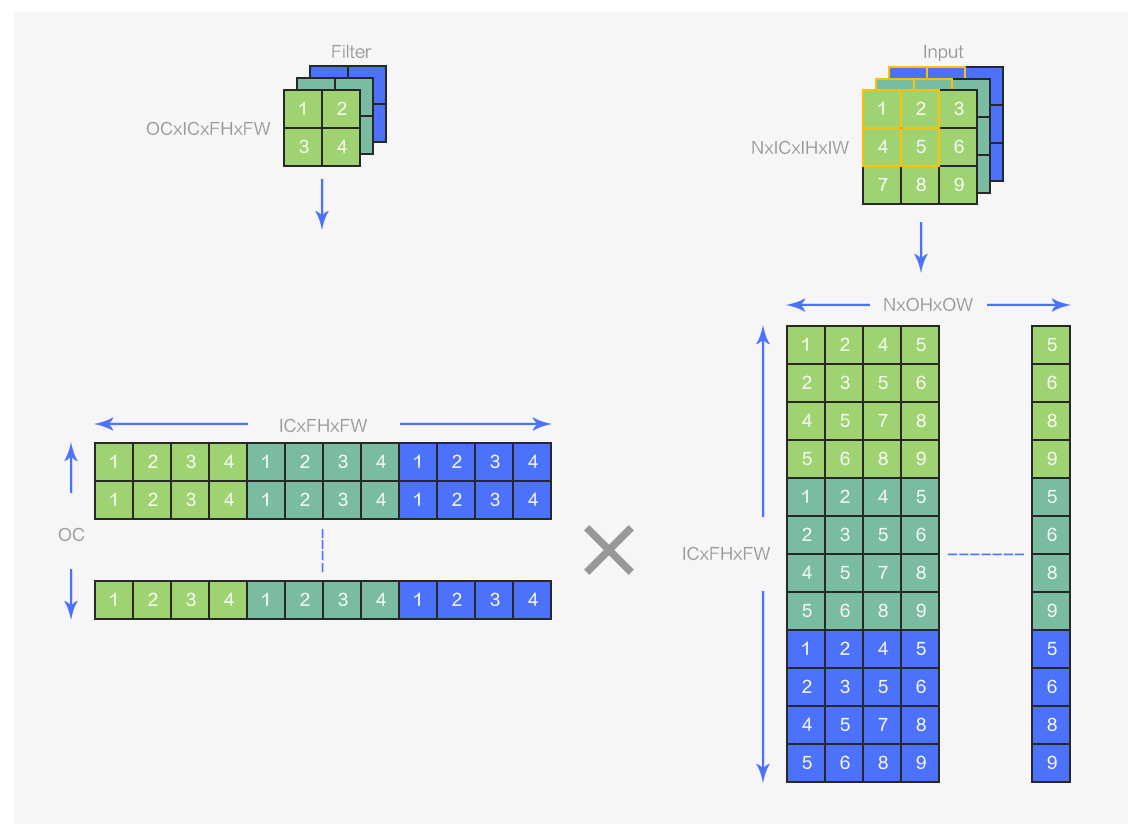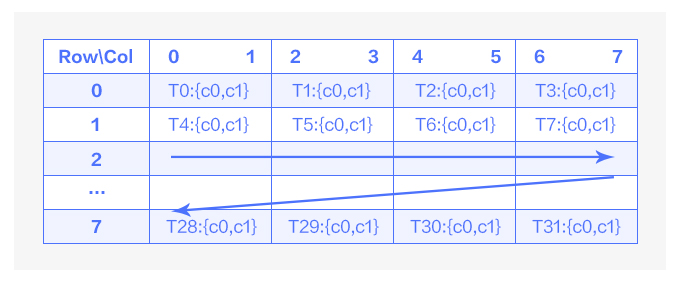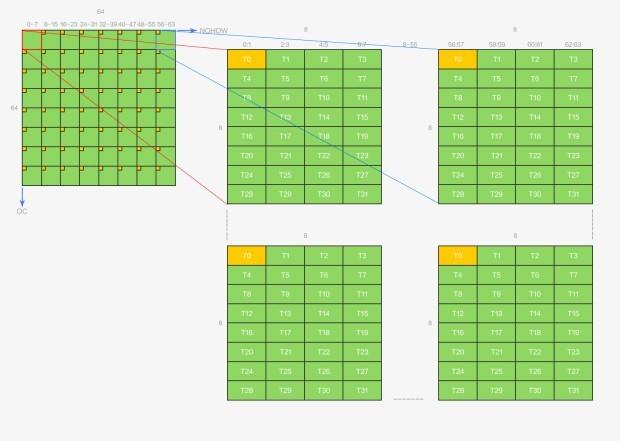• 每个线程中的数据都不连续，增大了数据处理难度，这些额外的处理计算可能会导致性能下降。
• 需要在纵向的 8 个线程间交换数据，会有同步的开销。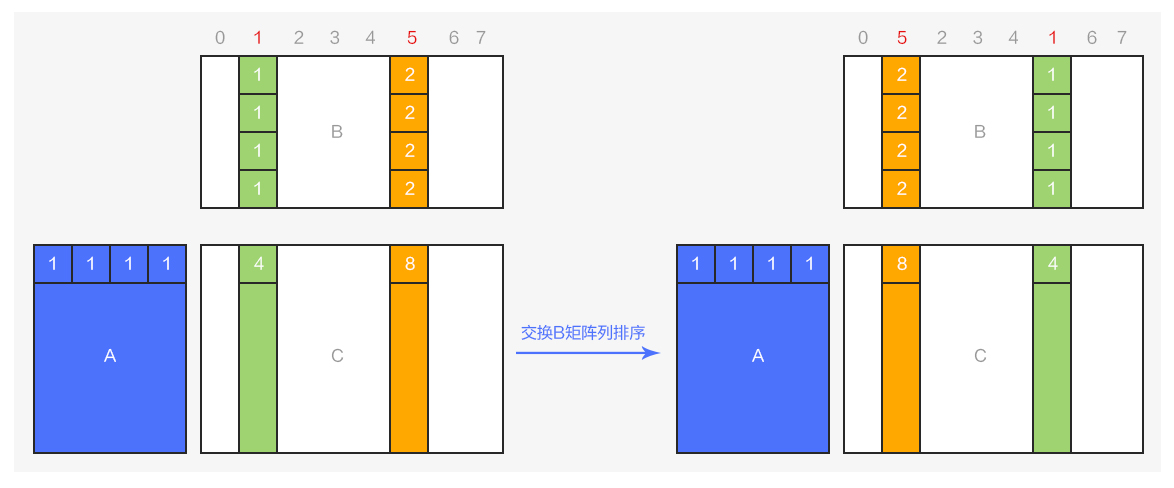• 将 OCxICFHFW 和 ICFHFWxNOHOW 的矩阵乘，变为 NOHOWxICFHFW 和 ICFHFWxOC 的矩阵乘，实现output 结果的转置，确保在 OC 维度上的数据连续，配合 NCHW64 的排布方式，便于将数据从寄存器上写回到 global memory 上。
• 通过对 ICFHFWxOC 矩阵的 OC 进行重新排序，实现 output 矩阵 NOHOWxOC 的 OC 维度和线程的对应关系更加合理，确保线程内部的数据连续性，避免线程间数据交换的开销。

## 总结&展望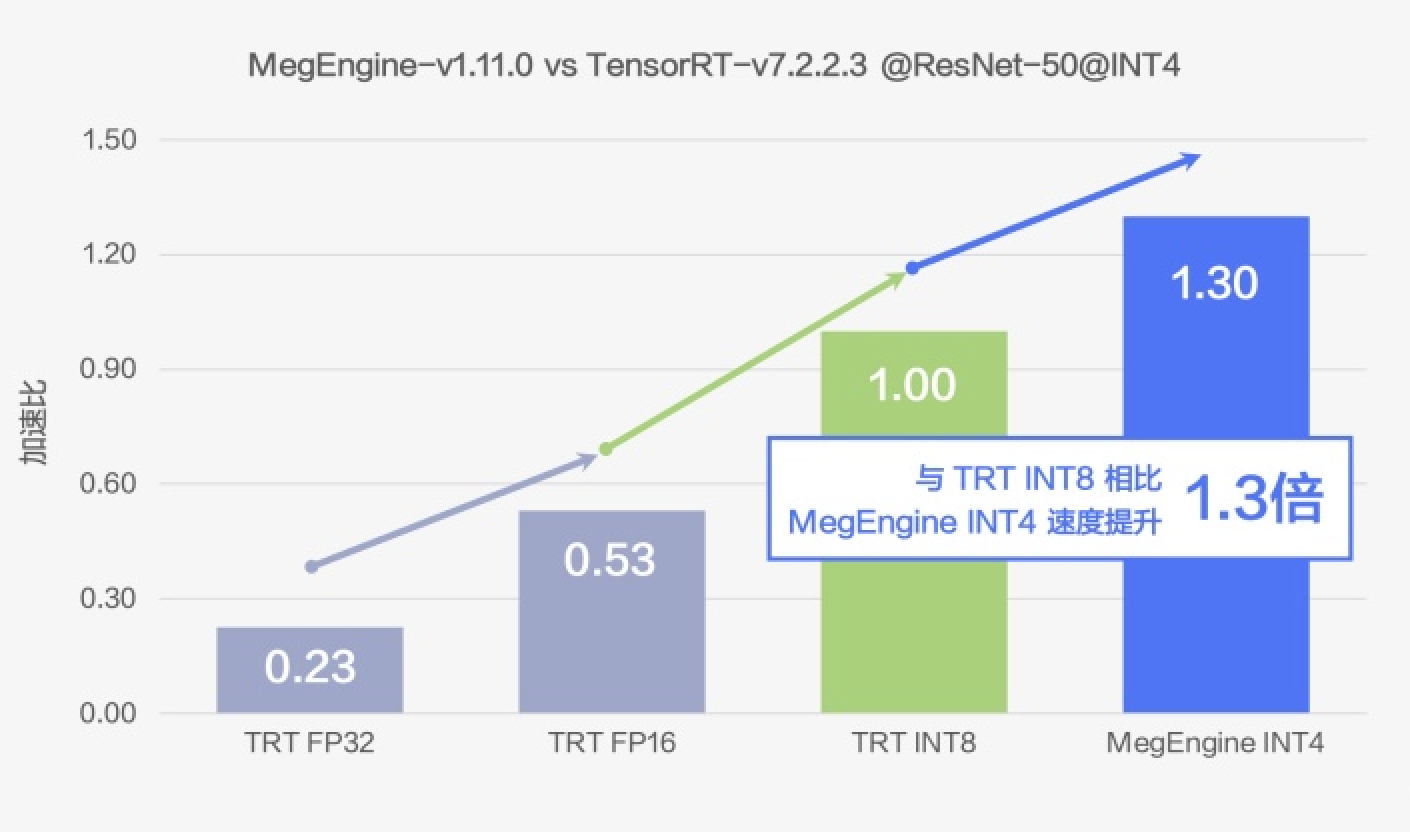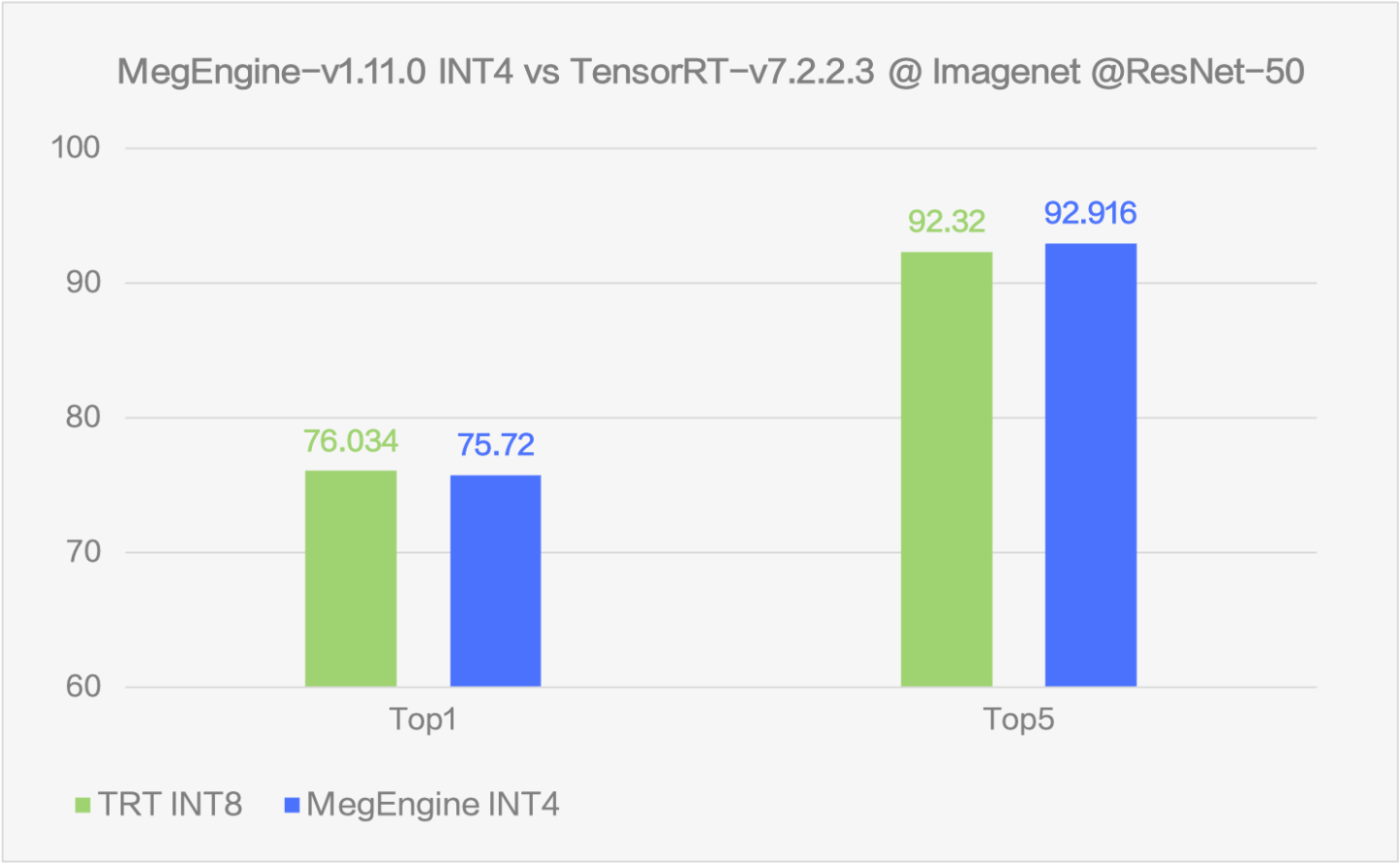## 附

GitHub：MegEngine 旷视天元 （欢迎 star~

Gitee：MegEngine/MegEngineTracedModule: 更友好的模型表示方案，模型训练到部署的桥梁相关推荐
• MegEngine 使用小技巧：量化

2023/04/25

• MegEngine TensorCore 卷积算子实现原理（上）

2021/05/18

• MegEngine TensorCore 卷积算子实现原理（下）

2021/05/18

• 深入理解混合精度训练：从 Tensor Core 到 CUDA 编程

2021/12/15

• CUDA 矩阵乘法终极优化指南

2021/09/14